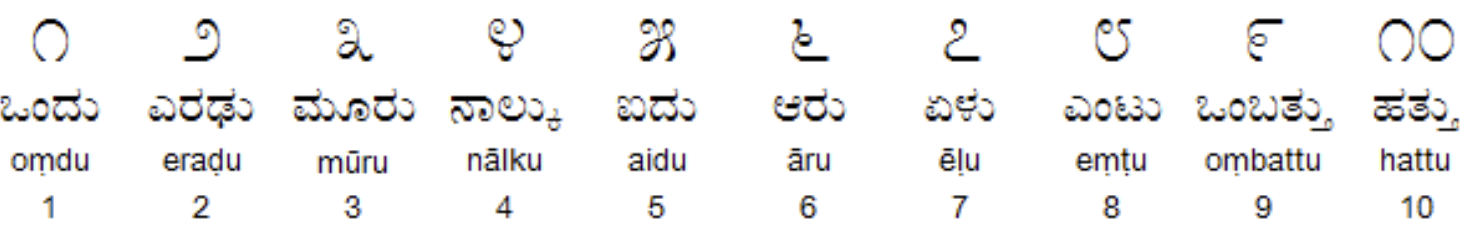# Kannada MNIST Prediction Classification using H2O AutoML in R

Kannada MNIST dataset is another MNIST-type Digits dataset for Kannada (Indian) Language. All details of the dataset curation has been captured in the paper titled: “Kannada-MNIST: A new handwritten digits dataset for the Kannada language.” by Vinay Uday Prabhu. The github repo of the author can be found here.The objective of this post is to demonstrate how to use h2o.ai’s automl function to quickly get a (better) baseline. Thsi also proves a point how these automl tools help democratizing Machine Learning Model Building process.

• h2o - for Machine Learning
• tidyverse - for Data Manipulation
library(h2o)
library(tidyverse)

### Initializing H2O Cluster

h2o::h2o.init()

train <- read_csv("~/Documents/R Codes/Kannada-MNIST/train.csv")
submission <- read_csv("~/Documents/R Codes/Kannada-MNIST//sample_submission.csv")

### Checking the shape / dimension of the dataframe

dim(train)

784 Pixel Values + 1 Label denoting what digit it’s.

### Label Count

train  %>% count(label)

### Visualizing the Kannada MNIST Digits

# visualize the digits
par(mfcol=c(6,6))

par(mar=c(0, 0, 3, 0), xaxs='i', yaxs='i')

for (idx in 1:36) {

im<-matrix((train[idx,2:ncol(train)]), nrow=28, ncol=28)

im_numbers <- apply(im, 2, as.numeric)

image(1:28, 1:28, im_numbers, col=gray((0:255)/255), main=paste(train$label[idx])) }### Converting R dataframe to H2O object which is required by H2O functions train_h <- as.h2o(train) test_h <- as.h2o(test) valid_h <- as.h2o(valid) ### Converting our numeric target variable into a factor for the algorithm to perform Classification train_h$label <- as.factor(train_h$label) valid_h$label <- as.factor(valid_h$label) ### Explanatory and Response Variables x <- names(train)[-1] y <- 'label' ### AutoML in Action aml <- h2o::h2o.automl(x = x, y = y, training_frame = train_h, nfolds = 3, leaderboard_frame = valid_h, max_runtime_secs = 1000) nfolds denotes the number of folds for cross-validation and max_runtime_secs represents the maximum amount of time the AutoML process can go on. ### AutoML Leaderboard Leaderboard is where the AutoML lists the top performing Models. aml@leaderboard ### Prediction and Submission pred <- h2o.predict(aml, test_h) submission$label <- as.vector(pred\$predict)

#write_csv(submission, "submission_automl.csv")

### Submission (for Kaggle)

write_csv(submission, "submission_automl.csv")

This is currently a playground Competition on Kaggle. So, this submission file can be submitted to this competition. Based on the above parameters the submission scored 0.90720 in the public leaderboard. 0.90 score in an MNIST Classification is close to nothing, but I hope this code snippet can serve as quick starter template for anyone attempting to begin with AutoML.